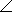# Electrical Engineering - RL Circuits

### Exercise :: RL Circuits - General Questions

6.

A 1.2 kresistor is in series with a 15 mH coil across a 10 kHz ac source. The magnitude of the total impedance is

 A. 152.6B. 1,526C. 1,200D. 942Explanation:

No answer description available for this question. Let us discuss.

7.

When the frequency is decreased, the impedance of a parallel RL circuit

 A. increases B. decreases C. remains constant D. is not a factor

Explanation:

No answer description available for this question. Let us discuss.

8.

A 470resistor and a 200 mH coil are in parallel. Both components are across a 1.5 kHz ac source. The total admittance of the circuit, in polar form, is

 A. 12.60–76° mS B. 12.1876° mS C. 218–76° mS D. 12.18–76° mS

Explanation:

No answer description available for this question. Let us discuss.

9.

Referring to Problem 23, the reactive power is

 A. 10 mVAR B. 100 mVAR C. 6.76 mVAR D. 148 mVAR

Explanation:

No answer description available for this question. Let us discuss.

10.

A 12 kresistor is in series with a 90 mH coil across an 8 kHz ac source. Current flow in the circuit, expressed in polar form, is I = 0.30° mA. The source voltage, expressed in polar form, is

 A. 3.8420.6° V B. 12.820.6° V C. 0.320.6° V D. 13.8469.4° V